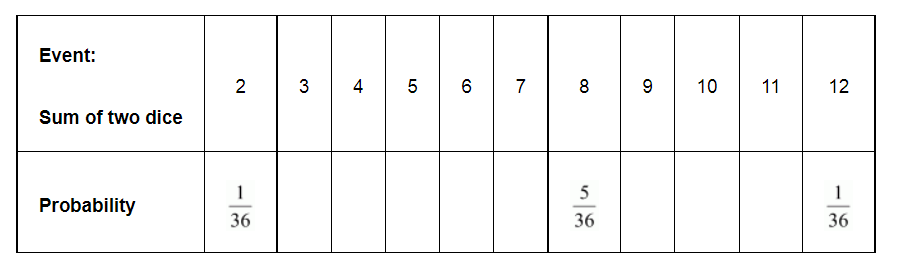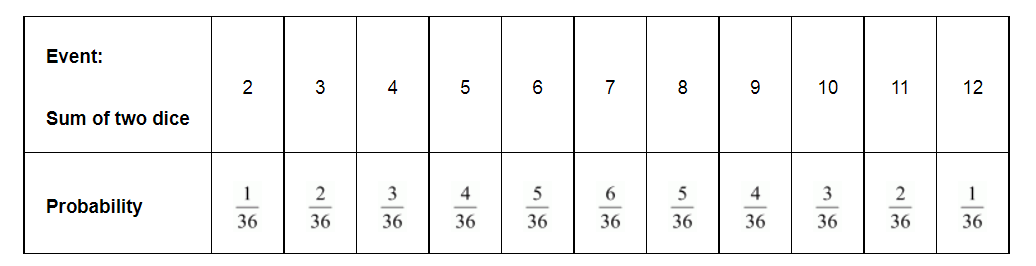# Two dice, one blue and one grey, are thrown at the same time.

Question:

Two dice, one blue and one grey, are thrown at the same time.

(i) Write down all the possible outcomes and complete the following table:(ii) A student argues that 'there are 11 possible outcomes $2,3,4,5,6,7,8,9,10,11$ and 12 . Therefore, each of them has a probability $\frac{1}{11}$. Do you agree with this argument?

Solution:

(i) It can be observed that,

To get the sum as 2, possible outcomes = (1, 1)

To get the sum as 3, possible outcomes = (2, 1) and (1, 2)

To get the sum as 4, possible outcomes = (3, 1), (1, 3), (2, 2)

To get the sum as 5, possible outcomes = (4, 1), (1, 4), (2, 3), (3, 2)

To get the sum as 6, possible outcomes = (5, 1), (1, 5), (2, 4), (4, 2),

(3, 3)

To get the sum as 7, possible outcomes = (6, 1), (1, 6), (2, 5), (5, 2),

(3, 4), (4, 3)

To get the sum as 8, possible outcomes = (6, 2), (2, 6), (3, 5), (5, 3),

(4, 4)

To get the sum as 9, possible outcomes = (3, 6), (6, 3), (4, 5), (5, 4)

To get the sum as 10, possible outcomes = (4, 6), (6, 4), (5, 5)

To get the sum as 11, possible outcomes = (5, 6), (6, 5)

To get the sum as 12, possible outcomes = (6, 6)(ii)Probability of each of these sums will not be $\frac{1}{11}$ as these sums are not equally likely.